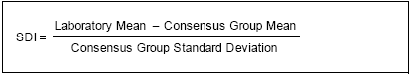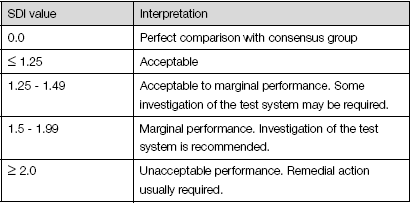##### Standard Deviation Index (SDI)

The standard deviation index is a measurement of bias (how close your value is to the target value). The Bio-Rad Unity™ Interlaboratory Program uses the consensus group value as the target value. Use the following formula to calculate the SDI:Interpreting the SDI

The target SDI is 0.0, which indicates there is not any difference between the laboratory mean and the consensus group mean. A SDI ±1 indicates a possible problem with the test.

The SDI expresses bias as increments of the standard deviation. A SDI of -1.8 indicates a negative bias of 1.8 standard deviations from the consensus group mean. This is not favorable.

Bias increases or decreases the percentage of patients outside the defined reference limit. For example, a positive bias decreases the percentage of patients normally outside the lower limit and increases the percentage of patients normally outside the upper reference limit. This creates an increase in false positive test results. Negative bias has an opposite effect and decreases true positives and creates false negatives.

Use the following guidelines to interpret the SDI: